# Chapter 19 Electricity Part 3 Electrical Resistance Ohms

• Slides: 45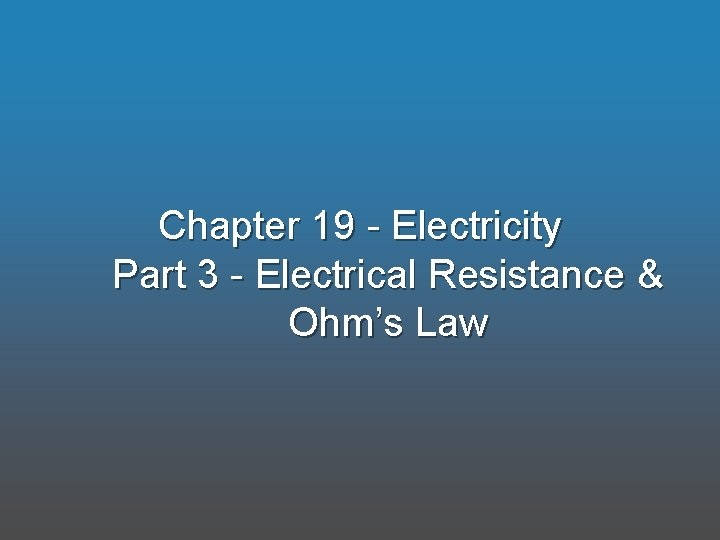Chapter 19 - Electricity Part 3 - Electrical Resistance & Ohm’s Law• In physics, what is resistance?• In physics, what is resistance? • Measure of how easily electric current moves through an object or electrical device. • Think of water flowing through a pipe or bottle opening.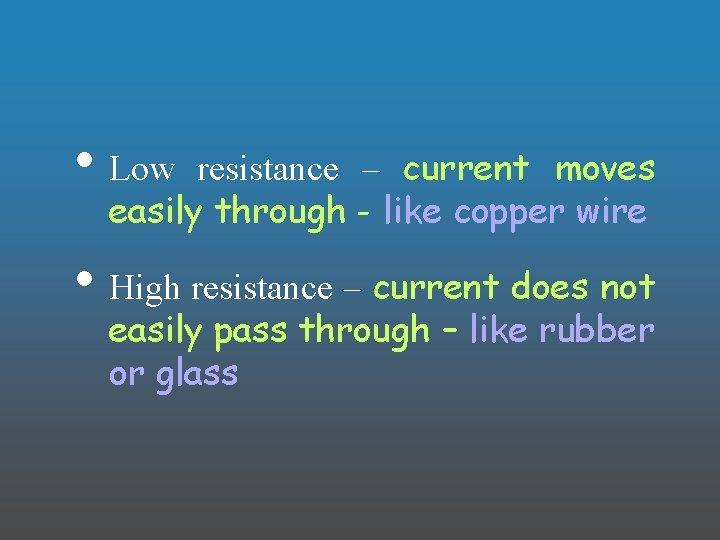• Low resistance – current moves easily through - like copper wire • High resistance – current does not easily pass through – like rubber or glass• How does changing the following properties of a wire change the resistance of the wire? • The length of the wire? ? • Thickness of the wire? ? • Temperature of the wire? ?• How does changing the following properties of a wire change the resistance of the wire? •• For a given voltage, the total amount of electrical resistance in a circuit determines. . . ? ? ? • Every device that uses electrical energy. . . ? ? ? • For a given voltage, . . . ? ? ?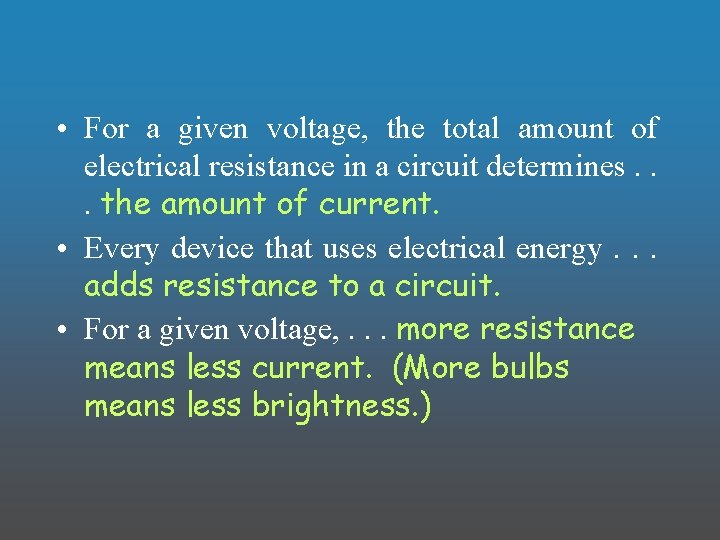• For a given voltage, the total amount of electrical resistance in a circuit determines. . . the amount of current. • Every device that uses electrical energy. . . adds resistance to a circuit. • For a given voltage, . . . more resistance means less current. (More bulbs means less brightness. )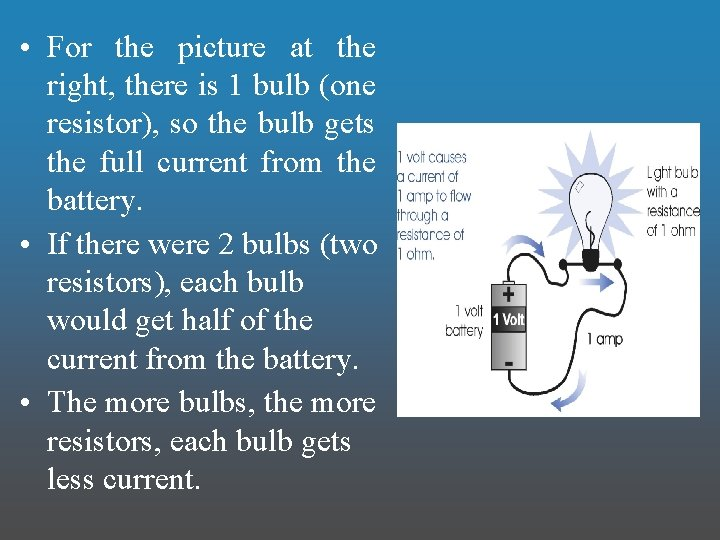• For the picture at the right, there is 1 bulb (one resistor), so the bulb gets the full current from the battery. • If there were 2 bulbs (two resistors), each bulb would get half of the current from the battery. • The more bulbs, the more resistors, each bulb gets less current.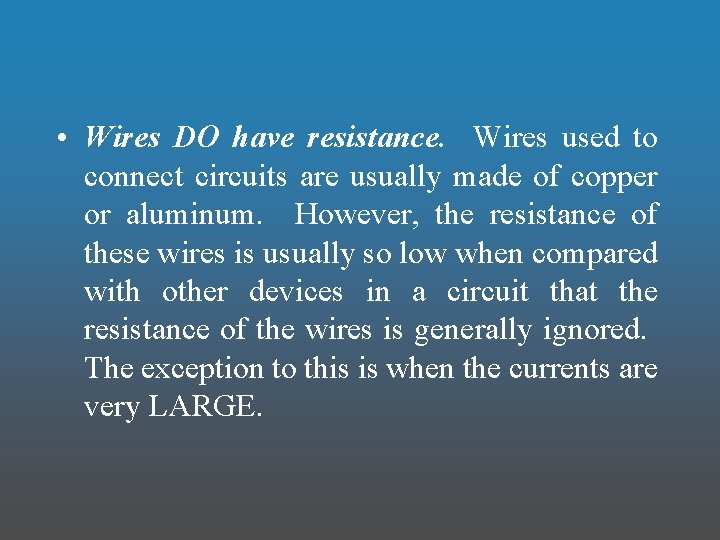• Wires DO have resistance. Wires used to connect circuits are usually made of copper or aluminum. However, the resistance of these wires is usually so low when compared with other devices in a circuit that the resistance of the wires is generally ignored. The exception to this is when the currents are very LARGE.• Ohm’s Law: relates current, voltage, and resistance in a circuit. • Current is. . . directly proportional to voltage and inversely proportional to resistance. • More voltage means ___? ? ? ___ current while more resistance means ___? ? ? ___ current.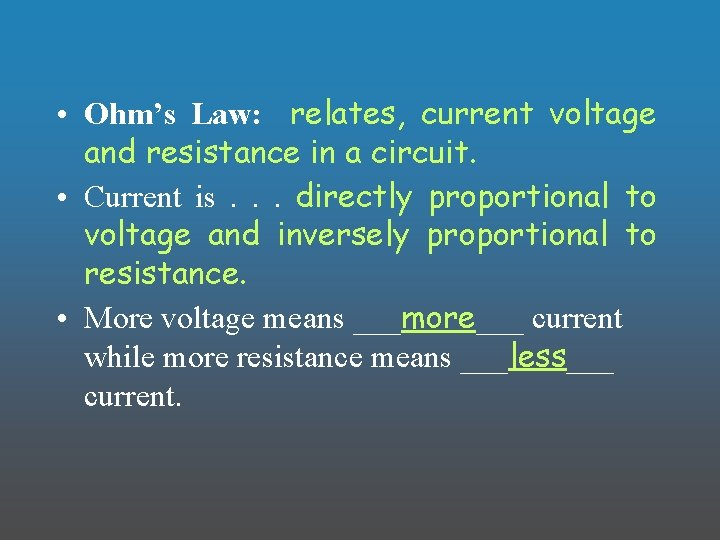• Ohm’s Law: relates, current voltage and resistance in a circuit. • Current is. . . directly proportional to voltage and inversely proportional to resistance. • More voltage means ___more___ current while more resistance means ___less___ current.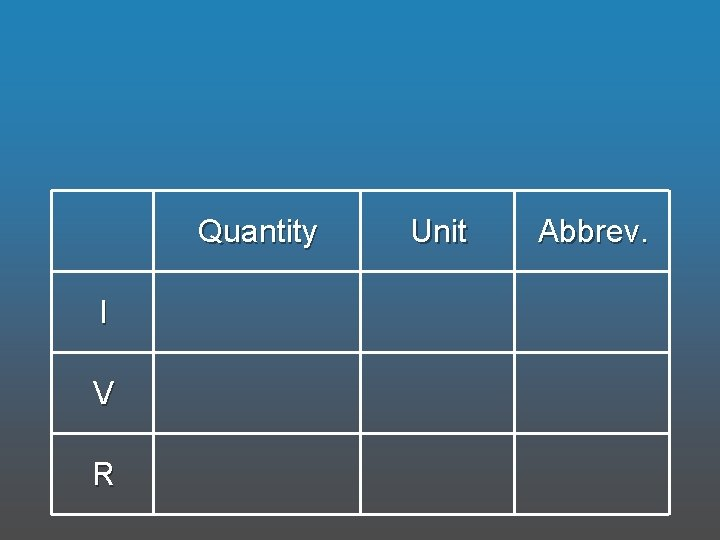Quantity I V R Unit Abbrev.Quantity Unit Abbrev. I Current Ampere A V Voltage Volt V R Resistance Ohm Ω• Every electrical device is designed with a specific resistance that allows them to draw the proper current when connected to the proper voltage. – Example: An electric motor needs 0. 75 amps. Design the motor with R = 2Ω so that when it is connected to a 1. 5 V battery, the motor draws the correct current.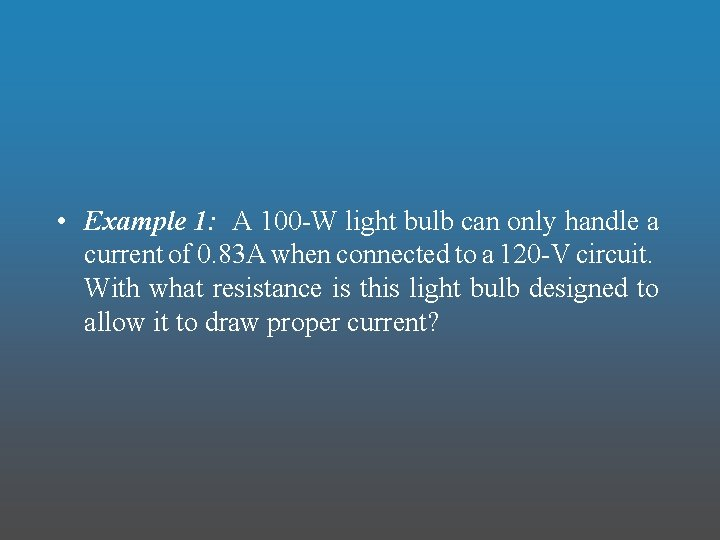• Example 1: A 100 -W light bulb can only handle a current of 0. 83 A when connected to a 120 -V circuit. With what resistance is this light bulb designed to allow it to draw proper current?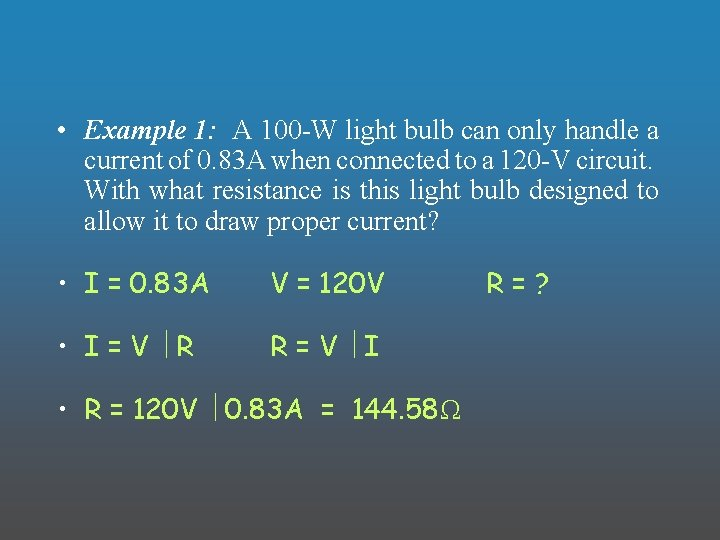• Example 1: A 100 -W light bulb can only handle a current of 0. 83 A when connected to a 120 -V circuit. With what resistance is this light bulb designed to allow it to draw proper current? • I = 0. 83 A V = 120 V • I=V÷R R=V÷I • R = 120 V ÷ 0. 83 A = 144. 58Ω R=?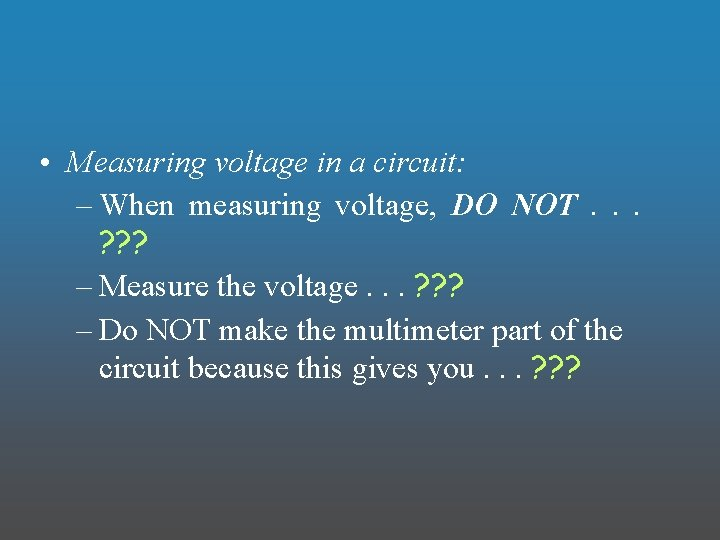• Measuring voltage in a circuit: – When measuring voltage, DO NOT. . . ? ? ? – Measure the voltage. . . ? ? ? – Do NOT make the multimeter part of the circuit because this gives you. . . ? ? ?• Measuring voltage in a circuit: – When measuring voltage, DO NOT. . . pass the current through the multimeter. – Measure the voltage. . . ACROSS the device you are interested in. – Do NOT make the multimeter part of the circuit because this gives you. . . the current.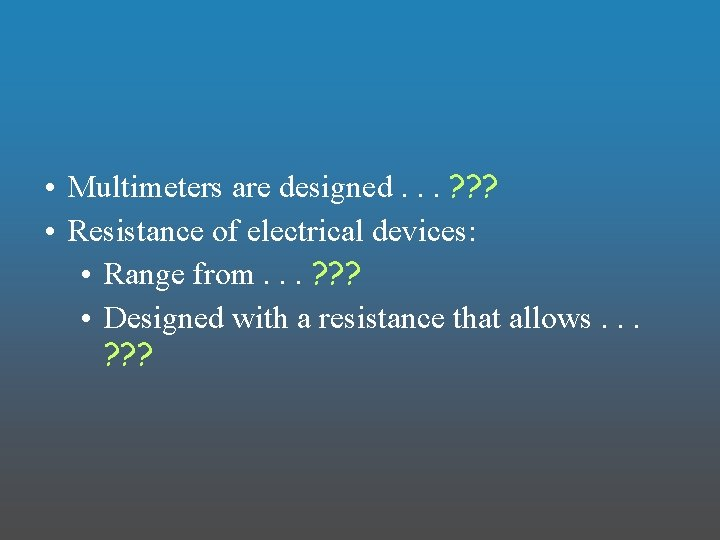• Multimeters are designed. . . ? ? ? • Resistance of electrical devices: • Range from. . . ? ? ? • Designed with a resistance that allows. . . ? ? ?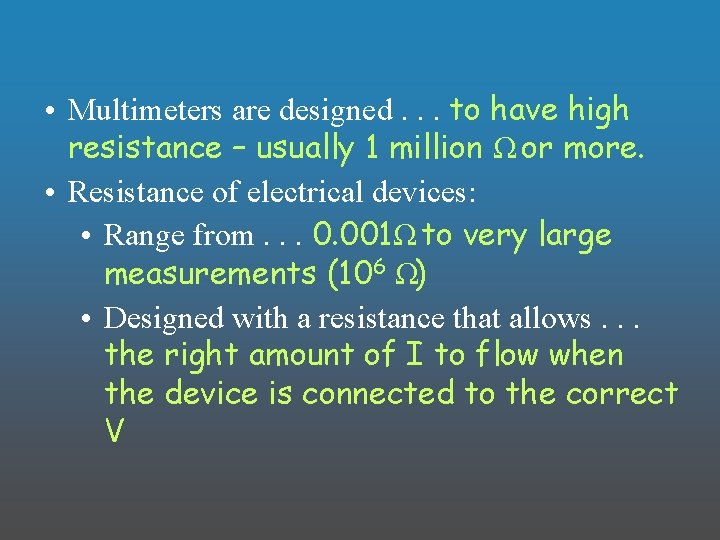• Multimeters are designed. . . to have high resistance – usually 1 million Ω or more. • Resistance of electrical devices: • Range from. . . 0. 001Ω to very large measurements (106 Ω) • Designed with a resistance that allows. . . the right amount of I to flow when the device is connected to the correct V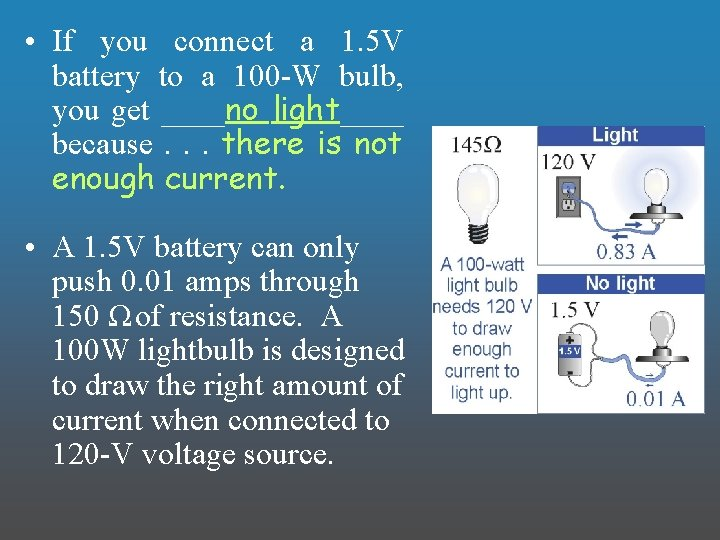• If you connect a 1. 5 V battery to a 100 -W bulb, you get ____no light____ because. . . there is not enough current. • A 1. 5 V battery can only push 0. 01 amps through 150 Ω of resistance. A 100 W lightbulb is designed to draw the right amount of current when connected to 120 -V voltage source.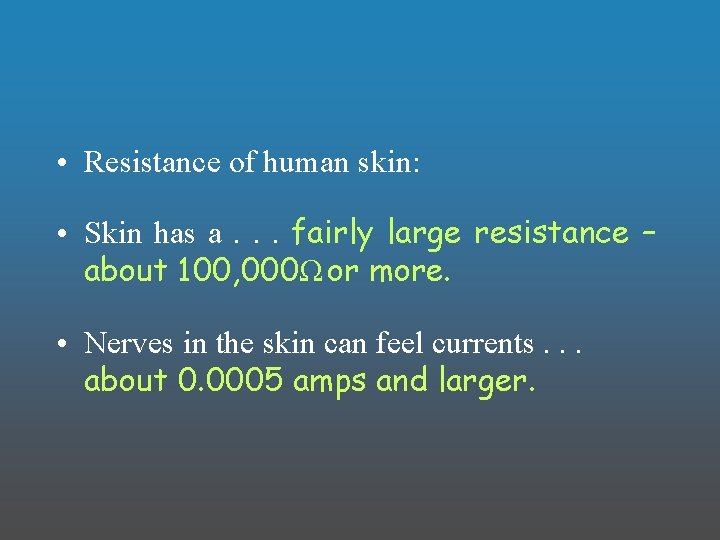• Resistance of human skin: • Skin has a. . . fairly large resistance – about 100, 000Ω or more. • Nerves in the skin can feel currents. . . about 0. 0005 amps and larger.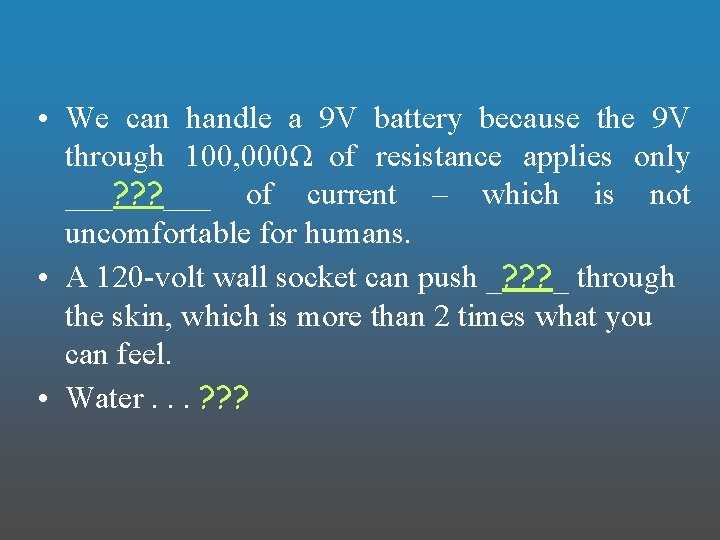• We can handle a 9 V battery because the 9 V through 100, 000Ω of resistance applies only ___? ? ? ___ of current – which is not uncomfortable for humans. • A 120 -volt wall socket can push _? ? ? _ through the skin, which is more than 2 times what you can feel. • Water. . . ? ? ?• We can handle a 9 V battery because the 9 V through 100, 000Ω of resistance applies only ___0. 00009 amps___ of current – which is not uncomfortable for humans. • A 120 -volt wall socket can push _0. 0012 amps_ through the skin, which is more than 2 times what you can feel. • Water. . . lowers the resistance of human skin so water and electricity are especially dangerous.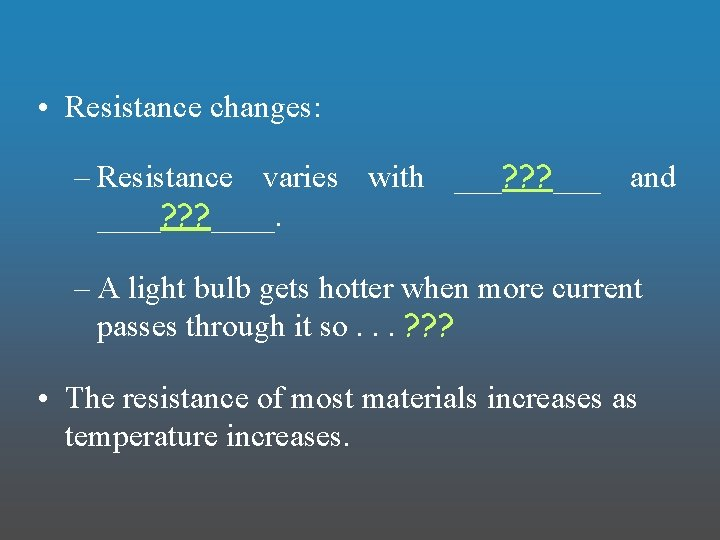• Resistance changes: – Resistance varies with ___? ? ? ___ and ____? ? ? ____. – A light bulb gets hotter when more current passes through it so. . . ? ? ? • The resistance of most materials increases as temperature increases.• Resistance changes: – Resistance varies with ___temperature___ and ____current____. – A light bulb gets hotter when more current passes through it so. . . its resistance increases with increased current. • The resistance of most materials increases as temperature increases.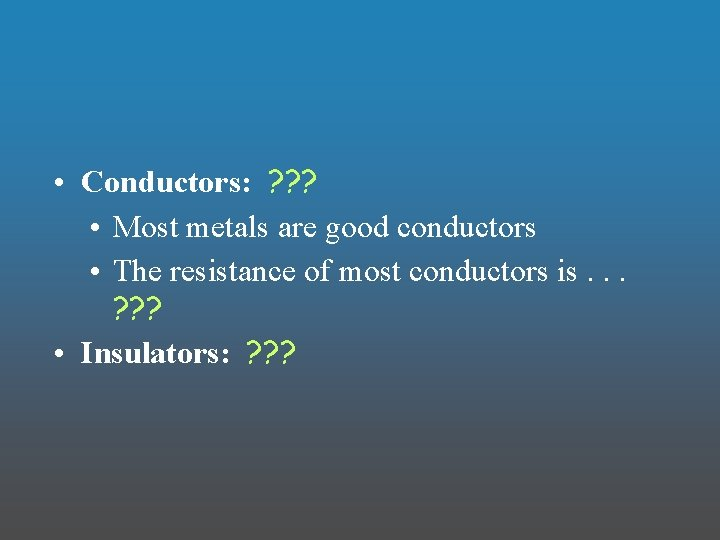• Conductors: ? ? ? • Most metals are good conductors • The resistance of most conductors is. . . ? ? ? • Insulators: ? ? ?• Conductors: allow electrical current to easily pass through – like copper • Most metals are good conductors • The resistance of most conductors is. . . low so that electric current may easily flow through them • Insulators: block the flow of current – like glass and plastic• Semiconductors: ? ? ? • Some amount of current will flow through all materials if. . . ? ? ? • Examples: ? ? ?• Semiconductors: neither a conductor nor an insulator • Some amount of current will flow through all materials if. . . enough voltage (electrical potential difference) is applied. • Examples: silicon, carbon, germanium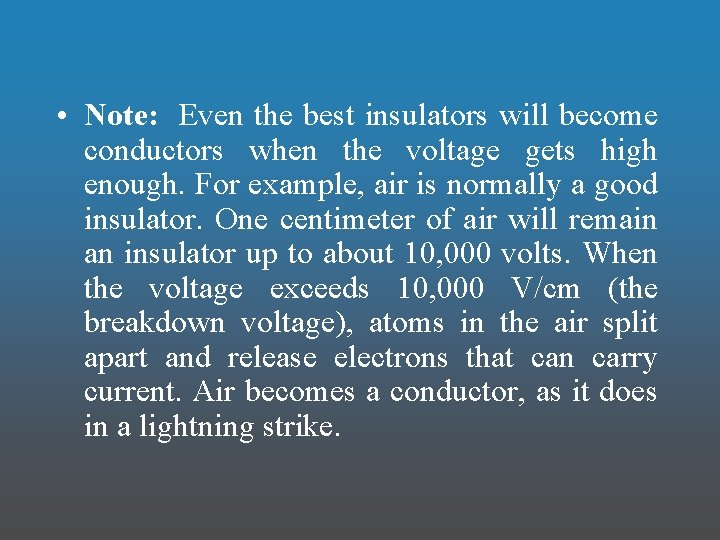• Note: Even the best insulators will become conductors when the voltage gets high enough. For example, air is normally a good insulator. One centimeter of air will remain an insulator up to about 10, 000 volts. When the voltage exceeds 10, 000 V/cm (the breakdown voltage), atoms in the air split apart and release electrons that can carry current. Air becomes a conductor, as it does in a lightning strike.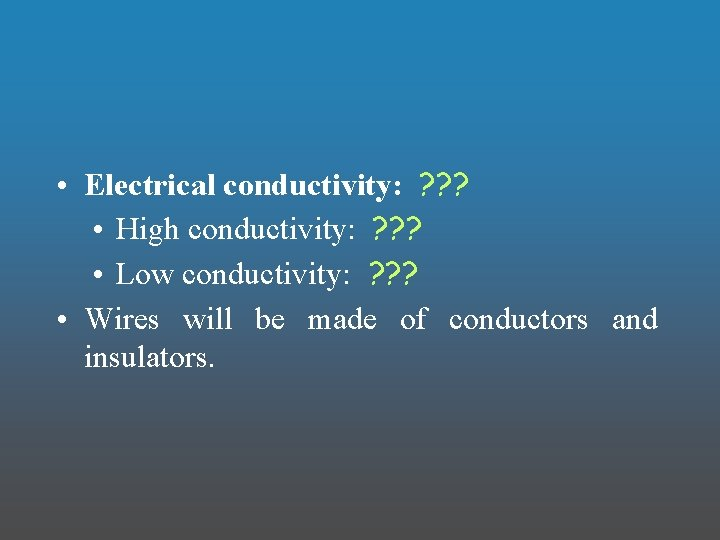• Electrical conductivity: ? ? ? • High conductivity: ? ? ? • Low conductivity: ? ? ? • Wires will be made of conductors and insulators.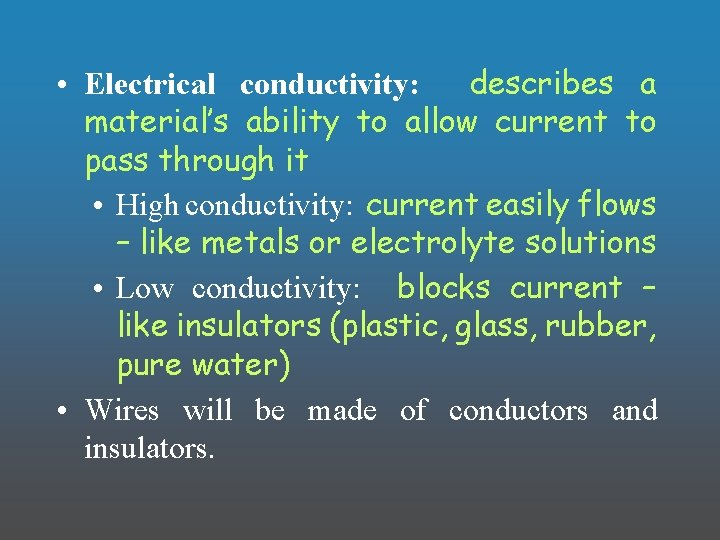• Electrical conductivity: describes a material’s ability to allow current to pass through it • High conductivity: current easily flows – like metals or electrolyte solutions • Low conductivity: blocks current – like insulators (plastic, glass, rubber, pure water) • Wires will be made of conductors and insulators.• Resistors: ? ? ? – Resistors are used to. . . ? ? ?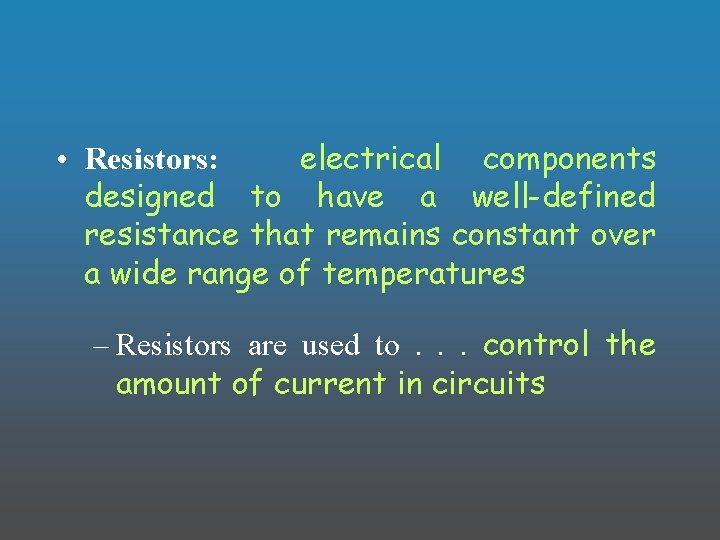• Resistors: electrical components designed to have a well-defined resistance that remains constant over a wide range of temperatures – Resistors are used to. . . control the amount of current in circuits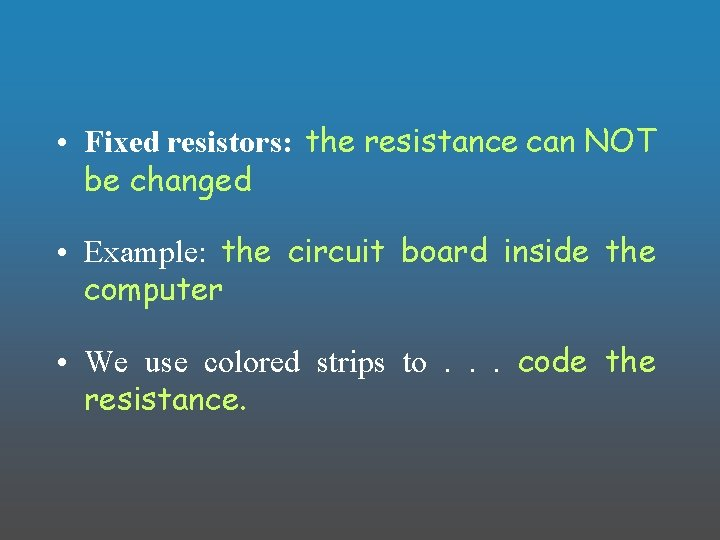• Fixed resistors: the resistance can NOT be changed • Example: the circuit board inside the computer • We use colored strips to. . . code the resistance.• The Resistor Color Code: • The first two stripes on a resistor tell the first two digits of its resistance. The third stripe tells you the power of 10 by which the first digits must be multiplied. The fourth stripe indicates the accuracy tolerance of the resistor. • The red and green stripes on the example resistor indicate that the first two digits are 2 and 5. The orange stripe means the 25 must be multiplied by 10 three times, for a total of 25, 000 Ω. The silver fourth stripe shows that the tolerance is +/ - 10%.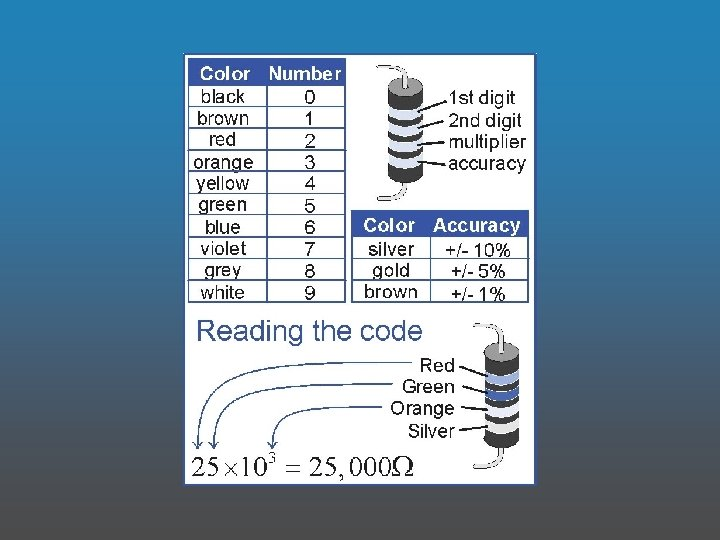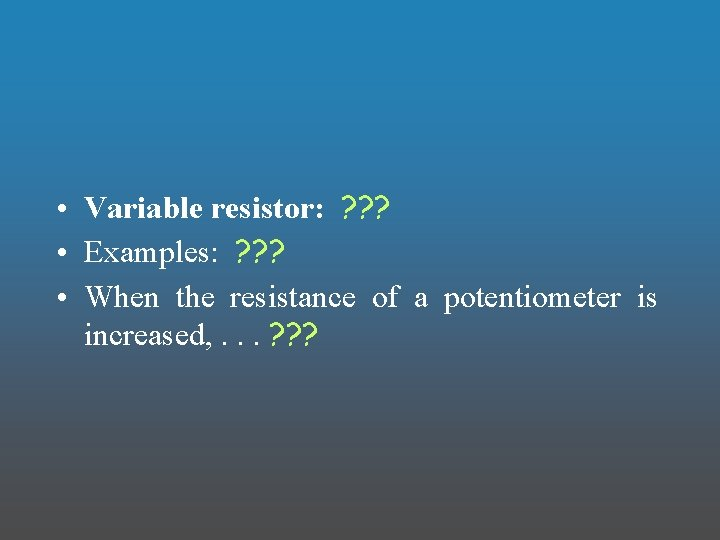• Variable resistor: ? ? ? • Examples: ? ? ? • When the resistance of a potentiometer is increased, . . . ? ? ?• Variable resistor: potentiometers – may be adjusted to have a range of resistances • Examples: dimmer switch or volume knob • When the resistance of a potentiometer is increased, . . . the current decreases (and the bulb is dimmed).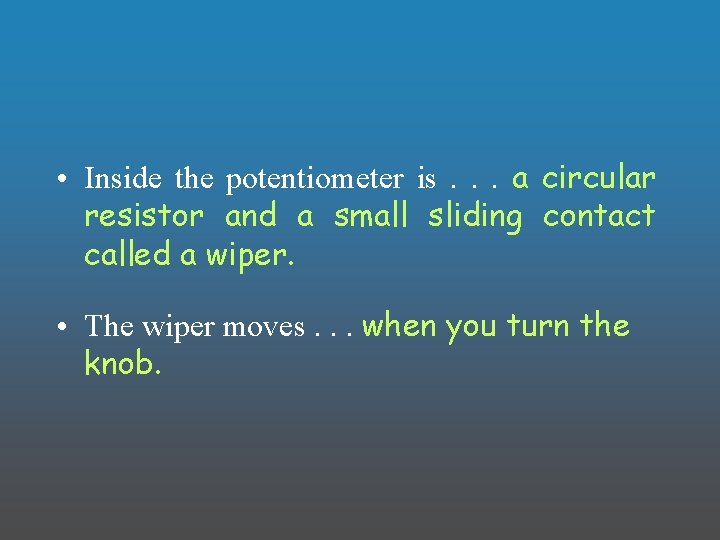• Inside the potentiometer is. . . a circular resistor and a small sliding contact called a wiper. • The wiper moves. . . when you turn the knob.• The End!!!